# Microbial Inactivation Kinetics and Heat Disinfection

Microbial Inactivation Kinetics and Heat Disinfection

Patrick J. McCormick

James J. Kaiser

Michael J. Schoene

Any discussion of the kinetics of the thermal destruction of microorganisms must acknowledge the defining contributions of individuals such as Rahn,1 Pflug,2 Holcomb and Pflug,3 Bigelow,4 Stumbo,5 Ball,6 Halvorson and Zielger,7 Russell,8 and many others. Their work laid the foundation for the development and validation of effective heat sterilization and disinfection treatments. Although heat processing has been used for centuries to reduce or eliminate microbial contamination and render items and goods safe for use or consumption, much of our knowledge of the kinetics of the thermal destruction of microorganisms and control of heat processing treatments has been derived from studies initially performed in the food industry during the first half of the 20th century. Stumbo5 describes how advances in canning, measurement, and processing technology developed concurrently and led to breakthroughs in our understanding of the kinetics of inactivation of microorganisms subjected to heat processing conditions. Many of the principles and concepts established during this time remain in widespread use today and have been incorporated into international pharmacopeias, disinfection, and sterilization standards.

Perhaps the greatest challenge in developing accurate models of the kinetics of heat inactivation is the microorganisms themselves. We can employ either homogenous populations of defined test organisms deposited on some form of carrier placed with or inoculated directly onto or within the product or goods being processed, or we can study the heterogeneous populations of microorganisms that are normally resident on the product (bioburden). The former approach is preferred when modeling heat inactivation kinetics because a hypothetical and homogenous population of microorganisms will respond in a more uniform manner to an applied heat stress as compared to a heterogonous population. Heterogeneous populations of microorganisms frequently consist of mixed genus and species in different states of growth or natural contamination with varying levels of heat resistance. This can complicate data analysis and lead to an inconsistent response to the applied heat stress as can studying the microorganisms in a different matrix native for the population. Most studies of applied heat processes are performed with a homogenous population of test organisms such as spores of Geobacillus stearothermophilus, Bacillus atrophaeus, Bacillus coagulans, Clostridium sporogenes, or others, many of which are commercially available and used in the form of biological indicators (BIs). The BIs are generally well characterized for their homogeneity and heat resistance as compared to product bioburden and are important tools in the development and validation of heat treatment processes (see chapter 65). In most circumstances, the use of a defined sterilization challenge in the form of a BI will provide a greater level of resistance to a given heat treatment than the bioburden that is typically present on or in the product and thus provide an additional margin of safety.9

As noted by Schmidt,10 “The only single practical criterion of the death of microorganisms is the failure to reproduce when, as far as is known, suitable conditions for reproduction are provided. This means that any organism which fails to show evidence of growth when placed under what is considered, in the light of our present knowledge of bacterial nutrition and growth requirements is considered as dead.” This statement is as true today as it was in 1954, even though our knowledge of the many different types of microorganisms has improved (eg, viruses). It underscores the fact that our knowledge and effective control of heat treatment processes rely primarily on our ability to recover and accurately enumerate microorganisms following exposure to heat treatment. This is critical to the development of effective heat treatment processes capable of attaining the desired level of microbial inactivation on a reproducible basis. Unfortunately, as anyone who has worked with microorganisms well knows, the recovery and enumeration of microorganisms is often subject to considerable variability. Although physical measurement
systems such as thermocouples, resistance temperature detectors, and pressure transducers can be calibrated to traceable standards with a high degree of accuracy, in most cases, this is not possible with viable microbiological systems. At best, the microbiological systems employed to document the efficacy of a given sterilization or disinfection process may be characterized as to their homogeneity and relative resistance to an applied heat treatment,11,12,13 but they cannot be calibrated to traceable physical standards.14 Thus, although calibrated physical measurement systems may be employed to accurately measure and control the critical parameters of an applied heat treatment process, the determination of the microbicidal efficacy of the process is often less precise. Studies regarding the thermal inactivation of microorganisms should therefore consider not only the inherent variability of the microorganisms themselves but also the variability associated with their enumeration and recovery. As discussed by Sutton,15 when considering bacteria and fungi, a plate count method often employed for the enumeration of microorganisms is subject to significant limitations such as growth abilities of the microorganisms present, accuracy, reproducibility, and the countable range per plate, as examples. Alternate microbiological enumeration techniques that rely on sophisticated technologies to detect the presence of viable microorganisms may also exhibit limited analytical capability because their performance is ultimately correlated to conventional microbiological methods.16,17 There also exists the specter of viable but nonculturable microorganisms that may not exhibit growth under the laboratory conditions tested but may remain capable of further reproduction when introduced into a suitable host.18 Further compounding this situation is that many studies have documented a wide range of variables that may impact the recovery of heat-stressed microorganisms. These must be controlled to the greatest extent possible to generate reliable data. Ultimately, as noted by Sutton,15 any quantitative measurement of microorganisms using conventional or alternative microbiological methods is at best an estimate of the true population of microorganisms that may be present in any given sample at any given time. On initial consideration, this would suggest that studies of the thermal inactivation of microorganisms could be fraught with error. A review of the literature indicates, however, that there are numerous studies documenting controlled and reproducible microbial inactivation kinetics across a broad range of conditions. Although these studies reflect the diligence of the investigators in controlling the many variables associated with microbiological testing and the recovery of injured microorganisms, they also reflect the fact that the raw data is frequently subject to logarithmic transformation based on the semilogarithmic model of the inactivation of microorganisms. Logarithmic transformation has the effect of both normalizing the distribution of errors in the data19 and stabilizing the variance.20 Indeed, the semilogarithmic model of microbial inactivation is the cornerstone of contemporary sterilization processing as a plot of the logarithm of the number of surviving microorganisms versus the duration of exposure or applied dose frequently yields a straight line. The selected processing conditions can then be extrapolated to the desired level of probability of microbial recovery. This model requires an acceptable degree of correlation or “goodness of fit” between the applied treatment and the resulting microbiological data, although this may be difficult to rigorously define from a statistical perspective, and the microbiological data may only span a limited range.THE SEMILOGARITHMIC SURVIVOR CURVE MODEL

As can be seen in Figure 11.1, when microbial inactivation data are plotted on a linear scale versus the duration of treatment under constant conditions, the resulting curve is of an exponential nature with an asymptotic decrease in the number of surviving microorganisms as the duration of exposure increases. Although it is evident that a greater number of microorganisms are being inactivated the longer the duration of the applied treatment, the information generated is of limited utility. As the number of surviving microorganisms decreases with the duration of exposure, resolution is lost; thus, it is difficult to use this data to extrapolate to a level of probability of a surviving
microorganism and thereby establish effective and reproducible treatment conditions. When the logarithm of the number of surviving microorganisms is plotted versus the duration of treatment under constant conditions, the effective population range that may be visualized is expanded and a linear relationship between the population of surviving microorganisms and the duration of treatment can become apparent (Figure 11.2). This information is far more useful as a linear relationship between microbial inactivation and the duration of the applied treatment or dose because it allows one to extrapolate to the desired probability of a surviving microorganism as appropriate to the intended application (Figure 11.3). This is of course an oversimplification because there are many factors involved in generating accurate and reproducible semilogarithmic inactivation curves of this nature, but it does serve to illustrate the primary attribute on which successful heat treatments for sterilization and disinfection are based; namely, the semilogarithmic nature of microbial inactivation.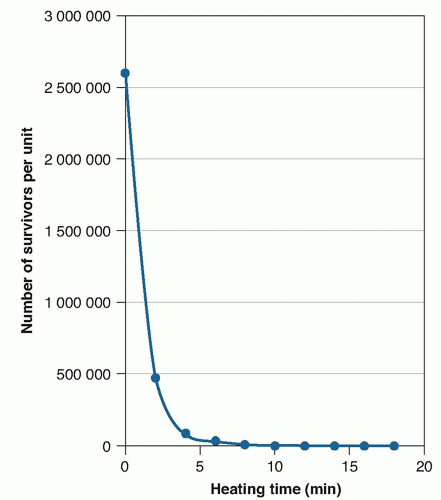FIGURE 11.1 Microbial survivor curve data plotted on an arithmetic scale, number of survivors per unit versus heating time.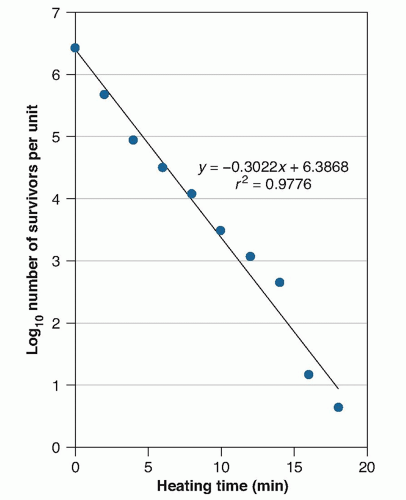FIGURE 11.2 The same microbial survivor data as per Figure 11.1, plotted on a semilogarithmic scale, with the number of survivors per unit plotted on the log scale on the y-axis versus the heating time on the arithmetic scale on the x-axis.

The mathematical basis for the semilogarithmic inactivation of microorganisms follows from the work of Rahn.1 Rahn1 observed that, in contrast to multicellular organisms, bacteria exposed to lethal agents were inactivated at a nearly constant rate, whereas multicellular organisms exhibited an initial lag followed by concave downward survivor curves. When mosquito larvae were immersed in heptylic acid or tadpoles heated at 35.5°C, no effect was apparent during the initial stages of treatment followed by an increase in the death rate to a maximum level, with a gradual decline in the rate of kill as the few remaining resistant organisms were killed off. With unicellular organisms, such as spores of Bacillus anthracis exposed to mercuric chloride or yeast of Torula monosa heated at 50°C, there was little or no lag apparent and inactivation occurred in a progressive geometric manner. For example, if one-half of the total population of bacteria or yeast were killed off in the first minute of exposure, one-half of the remaining population would be killed off in the second minute of exposure leaving one-fourth of the initial population alive at the second minute, etc. By plotting the logarithm of the surviving population of bacteria or yeast versus the time of exposure (or dose) of the lethal agent, a straight line was apparent. Based on the law of mass action, Rahn1 attributed the logarithmic order of death of bacteria to the inactivation of a single critical molecule within the cell. This was analogous to the single hit theory of inactivation as proposed by Chick21 for chemical disinfectants based on a first-order chemical reaction.FIGURE 11.3 Extrapolation of the data of Figure 11.2 to a sterility assurance level (SAL) of 10-6 or a one-in-a-million probability of a surviving microorganism.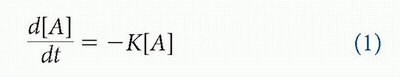For sterilization and disinfection processes, the rate of this inactivation can be expressed as the following differential equation: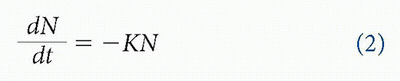where N is the number of microorganisms surviving after treatment time (t) and K is a reaction-rate constant, which for thermal treatments is a function of temperature. A negative term is assigned to the right side of the equation to reflect the inactivation of microorganisms with continued treatment. Integrating this equation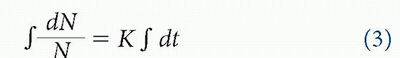One can then evaluate the constant C using the boundary conditions. At time zero, C becomes the initial population, which is N0. The equation thus becomes:Converting this equation from natural logarithms of base e to logarithms of base “10”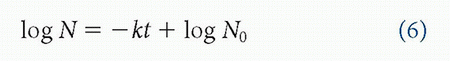Note that in the base 10 system, the lower case term k is used to replace the term K; the two constants are related in that k = 0.4343 K.

Now that the differential equation for first-order reaction kinetics has been converted to a form that is relatively user friendly, one must also consider the treatment of time in this process. As the rate of microbial inactivation is not necessarily related directly to the sterilizer clock time due to lag factors and other considerations, the term t is replaced with the term U, which is the equivalent minutes at the test temperature. The equation then becomesWe must now consider the D-value, or decimal reduction time, which is generally defined as the time or dose required to reduce a population of microorganisms by 1 log (90%) under a stated set of conditions. This is a useful measure of the resistance of a given population of microorganisms to a heat treatment process and is frequently employed to define a minimum acceptable level of sterilization such as a 12D reduction in a population of test organisms or bioburden.22 Although a D-value (also known as a D10 value) can be determined in several ways, it is perhaps most easily visualized as the exposure time or dose required for the survivor curve to traverse 1 log cycle. From equation (7), we see that if log N is plotted versus the equivalent exposure time U, a straight line is obtained with slope –k (Figure 11.4). By definition, the slope of a straight line is the tangent of the angle θ, which is expressed as (Δlog NU). Examining the values of Δlog N and ΔU in Figure 11.4, we find that the numerator in the slope equation (Δlog NU) when log N2 – log N1 are 1 log apart has a negative slope and is always – 1. For example, log 10 – log 100 = 1 – 2 = – 1. For the denominator in the slope equation for a 1-log change in N, the value of U is U2U1, which is ΔU. Therefore, for a 1-log change in N, the slope is – 1/ΔU.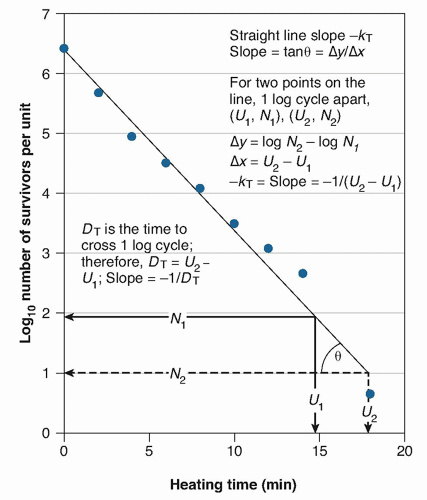FIGURE 11.4 Graph illustrating the relationship between D-value and slope of the survivor curve on a semilogarithmic graph. Based on Pflug et al.23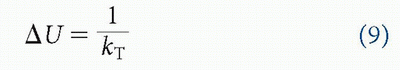Over time, the use of the reaction rate constant k was replaced with the use of the term D or D-value, which is the time at temperature T for the straightline semilogarithmic survivor curve to traverse 1 log cycle (a 90% reduction in N) as discussed earlier. Because the rate of microbial inactivation will vary with the temperature of the heat stress applied, the D-value is usually denoted as the term DT to denote exposure to constant temperature conditions (eg, D121°C). Substituting the term DT for the term kt in equation (7) based on the equivalency of these terms as expressed in equation (9), we have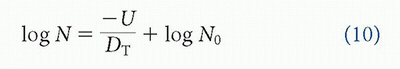As can be seen from this equation, the D-value can also be defined as the negative reciprocal of the slope of the semilogarithmic survivor curve. Rearranging the terms of the earlier equation, a more useful equation for the determination of the D-value based on plate count data generated from a semilogarithmic survivor curve is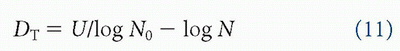One of the issues that is frequently encountered when modeling semilogarithmic inactivation kinetics is that even though most of the curve is of a linear nature, during the initial phases of exposure, shoulders that are concave either upward or downward may be apparent and/or tailing may occur, which can skew the fit of the model (Figure 11.5). Several factors can explain this departure from linearity, including multiple hit as opposed to single-hit inactivation kinetics,24 cell clumping,1 mixed populations of cells of varying ages,1 spore dormancy,25 variations in the applied heat treatment, poor control over experimental variables, presence of interfering factors, etc. Note that the linearity of the survivor curve is a function not only of the homogeneity of the population of microorganisms being tested but also of one’s ability to control the applied heat treatment within a narrow temperature band. Where variations from linearity occur during the initial phases of heating such as those indicated for curves B and D in Figure 11.5, it may be acceptable in some circumstances to ignore the N0 data and construct a survivor curve based on the remainder of the data. A better means to reconcile the deviation from linearity during the initial phases of heating is to modify equation (10) by incorporating an intercept ratio (IR), which is defined as log Y0/log N0 where Y0 is the intercept of the curve with the y-axis and N0 is the initial population of microorganisms. The resulting equation will take the following form:FIGURE 11.5 Shape of semilogarithmic survivor curves: straight line through the initial number, N0 (curve A); concave downward (curve B); concave upward (curve C); activation effect (curve D); sigmoid (curve E); and a straight line with a tail (curve F). Based on Pflug et al.23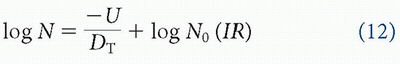Thus, for survivor curves with an IR value >1.0, the curve will be concave downward (see Figure 11.5, curve B), whereas those with an IR value <1.0 will be concave upward (see Figure 11.5, curve C). Survivor curves with an IR value of 1.0 will obviously be straight lines through N0 (see Figure 11.5, curve A). Holcomb et al26 have also suggested the use of an intercept index defined as log (y-intercept at U = 0 / N0) in order to address deviations from linearity in the initial phases of the semilogarithmic survivor curve. Although it is possible to use different mathematical approaches to assess the goodness of fit of the data, visual inspection of the survivor curve remains one of the easiest means to evaluate its linearity.

In addition to the shoulders that may be present in the survivor curve during the initial heating phase of the process, one must also consider how well the data from the linear portion of the curve fits the resulting model. A best fit estimate of the data can be readily determined using a graphing function or statistics package with a resulting coefficient of determination (r2) of not less than 0.8 (eg, as defined for heat-based BIs in ISO 11138-1). Although this is acceptable for many applications, it is not necessarily the most rigorous approach because the range over which it is applied is not linked to a maximum degree of uncertainty. The least squares method of Draper and Smith27 may also be employed to estimate the best fit of the data to a straight line as discussed by Pflug et al.23 The least squares method makes the following assumptions: (1) the true model describing the relationship between the logarithm of the number of surviving organisms and heating time is a linear one, (2) the deviation in the log number of survivors from the straight line is a random variable with expected value of zero, (3) the expected deviation is the same for all heating times, and (4) the deviations for different heating times are uncorrelated. The following model is employed:where yu = log NU the logarithm of the number of survivors at time U; U = equivalent heating time at the test temperature; α = the y-intercept at U = 0; β = slope of the line; and ε = deviation component, a random variable with mean zero, constant variance equal to some value σ2, and which is uncorrelated for different heating times.

If n observations have been made at various heating times (U1, U2, … Un), not all necessarily distinct, we can derive estimates of α and β, which are denoted asand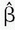. This is done by choosing α and β to minimize the following sum of squared deviations: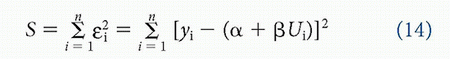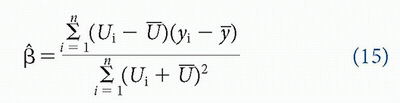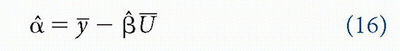whereAn unbiased estimate (s2) of the variance σ2 of the random variable ε is given by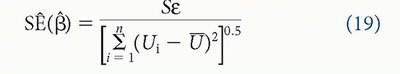Equations (14), (15), (16), (17), (18) and (19) are defining equations and can be expanded and put into a simpler form for computation.

Because there are estimates of α and β and standard errors of those estimates, we can form confidence intervals (CIs) for the two parameters. The 95% CIs based on our experimental data would be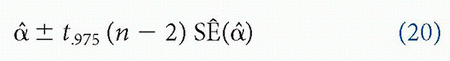andwhere t.975 (n – 2) is the 0.975 percentage point of the Student’s t distribution with (n – 2) degrees of freedom.

These CIs for the intercept and slope parameters have the following interpretation in our model: If an experiment is carried out many times in exactly the same way, then we would expect the CIs calculated from each set of data to cover the true values of α and β approximately 95% of the time.

An estimate for the parameter D, defined in equation (10), can be obtained from the following equation:The estimate in equation (22) is called the quasi-least squares estimator of D because the minimization of the sum of squares in equation (14) was with respect to β = -1/D, and not D. McHugh and Sundararaj28 described various methods for estimating D, including quasi-least squares and the weighted and unweighted least squares methods, and the method of maximum likelihood.

An approximate 95% CI for D is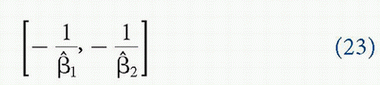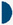NONLINEAR SURVIVOR CURVES

The phenomenon of nonlinear survivor curves has been attributed to a number of potential causes including clumping of microorganisms, population heterogeneity, differences in heat resistance within the population,29,30 increase in heat resistance during the heating process,31 activation (germination) at high temperatures of a normally dormant spore population,32 and various other causes. Although there has been much speculation regarding the potential causes, it should be remembered that nonlinear survivor curves may be simply caused by either poor control over the heat treatment process or issues associated with the laboratory analysis phase and the enumeration of surviving microorganisms. Nonlinear behavior can occur with any sterilization or disinfection process.33 To address this, a number of alternative models to the classic first-order inactivation kinetics have been proposed for the heat inactivation of bacteria. For example, van Boekel34 discussed the application of the Weibull distribution model to address the nonlinearity of observed semilogarithmic inactivation curves of vegetative cells subjected to heat treatment. As opposed to assuming that the inactivation of microorganisms is a consequence of a single lethal event, the Weibull approach considers lethal events as probabilistic events with the resulting survivor curve being a cumulative form of the individual inactivation time of each cell. As described by van Boekel34 and Head and Cenkowski35 in the Weibull model, the distribution of different individual inactivation times takes the following form: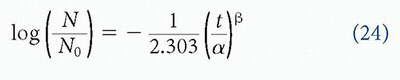Where: N = number of survivors at time t

N0 = initial number of microorganisms

t = treatment time

α = scale parameter (typically minutes or seconds)

β = shape parameter (Weibull slope)

If the resulting semilogarithmic plot is linear, β = 1.0, whereas if β >1.0, the curve is concave downward and concave upward if β <1.0. The 90th percentile of the failure time distribution, tR, which is also referred to as the reliable life in failure analysis (roughly analogous to the D-value when 1 log reduction is concerned), may be calculated asThe Weibull model has been successfully employed by a number of investigators to address nonlinear inactivation kinetics in various systems including vegetative cells heated in various menstruum,34 bacterial spores,35,36,37 and heat-resistant fungi.38,39,40 Other models include the shoulder/tail model discussed by Geeraerd et al41 that incorporates an initial shoulder followed by an exponential inactivation phase and subsequent tailing phase, the Gompertz model that also addresses shoulders and tailing of the survivor curve,42,43,44 and the biphasic model for which there are two different inactivation slopes (eg, assuming two distinct subpopulations of microorganisms29,45). Many of these models have been developed to address the kinetics of inactivation associated with the thermal treatment of foods and juices under low-temperature processing conditions as well as combination treatments.46 Although these models are very useful and may indeed provide a better understanding of the behavior of a population of microorganisms when subjected to a particular stress or combination of stresses, it is likely that the semilogarithmic model will remain predominant for the foreseeable future due to its simplicity and ease of application.QUANTAL OR FRACTION-NEGATIVE ANALYSIS

As discussed earlier, the continued application of a controlled heat treatment to a homogenous population of microorganisms generally results in a predictable semilogarithmic order of inactivation that can be readily visualized by plotting the logarithm of surviving microorganisms versus the duration of heat treatment. This model is effective only to the limit of quantitation of plate count technology, which is approximately 25 to 250 colony-forming units (CFUs) for most microorganisms. As one would anticipate, counting error increases significantly the fewer the number of colonies present on a plate with an error of 20% as a percentage of the mean for 25 CFUs, 44.7% for 5 CFUs, and 100% for 1 CFU.47 Due to the increase in counting error associated with the loss of countable colonies as the heat treatment progresses, we must therefore rely on quantal or fraction-negative analysis, which is capable of measurements in the range of 5 to 0.01 microorganisms per unit (Figure 11.6). In quantal or fraction-negative analysis, replicate samples of the challenge microorganism are subjected to graded exposures of treatment under constant conditions and the number of samples surviving treatment qualitatively determined (growth or no growth) for each exposure period. This typically takes the form of culturing each set of replicate samples processed for a given exposure time in individual tubes of growth medium and determining the fraction of tubes positive or negative for growth of the test organism based on visible turbidity following incubation for a defined period of time. Each set that is processed may exhibit complete recovery or growth of all samples tested, partial (dichotomous) recovery of the samples tested, or complete inactivation of all samples tested with increasing duration of heat treatment.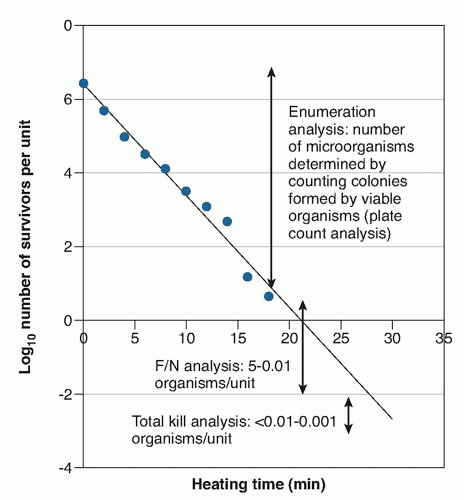FIGURE 11.6 Survivor curve illustrating the enumeration, fraction-negative (F/N, or quantal) measurement areas, and region where there is essentially total kill.

One of the earliest approaches to the analysis of quantal data was the thermal death time or end point analysis as described by Bigelow and Esty48 and Townsend et al.49 The thermal death time approach was initially employed in the canning industry to establish retort process times. This approach consisted of plotting the positive and negative results of replicate samples processed at various times and temperatures as a function of time on a logarithmic scale versus temperature (Figure 11.7). When any of the replicate units were positive, this was indicated with a plus sign. For those times when all replicates were negative, a zero was employed. The thermal death time for a given temperature was
assumed to be between the longest heating time when a positive unit was obtained and the shortest heating time when all the units were negative. A shortcoming of this approach is that it does not consider the initial inoculum level (N0) or the number of replicates per time point. Nevertheless, it was a reasonable if somewhat simplistic attempt at establishing effective process control based on the rate of inactivation of microorganisms under varying conditions. Today, the most common approach to the analysis of quantal data is to employ either the Stumbo-Murphy-Cochran method, which is based on most probable number (MPN) analysis, or the Holcomb-Spearman-Karber method, which is based on the mean time until sterility (UHSK) as indicated in ISO 11138-1.50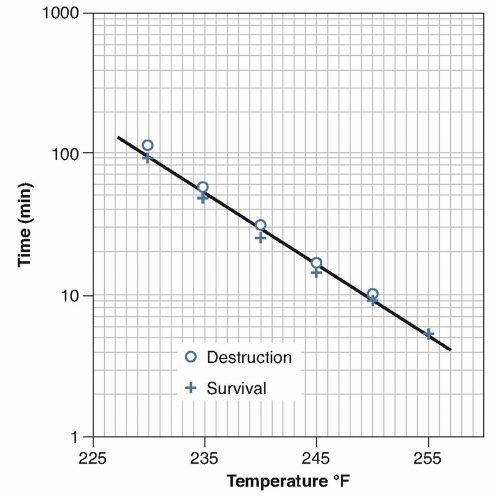FIGURE 11.7 Thermal death time curve for spores of PA 3679 in beef and gravy in thermal time cans. F0 = 9.5 min and z = 20°F. Based on Pflug.2

The Stumbo-Murphy-Cochran method is a modification of the semilogarithmic model expressed in equation (10). This is easily rearranged to express the D-value as follows:Where: Ui = a specific heating time

N0 = the initial number of microorganisms in each replicate unit

N = number of survivors at Ui

Stumbo et al51 proposed that an estimate of N (the number of survivors in the quantal region) could be based on the MPN equation of Halvorson and Zeigler7:Where: n = number of units heated

r = number of units negative for growth

The MPN equation is essentially the probability of the “no survivors term” of the Poisson equation P(0) = e-N. A dichotomous result in the quantal region occurs because of a probabilistic distribution of a few surviving microorganisms among replicate units. In fraction-negative testing when we have dichotomous results, the units that show no growth (the P units) are the critical units in the analysis because only in these units do we truly know the number of surviving organisms (zero) per sample because we cannot determine by inspection the number of surviving microorganisms at a given exposure time in those units that are positive (turbid) for growth. Table 11.1 provides an estimate of the number of surviving microorganisms (N) per unit calculated by the MPN method from 1 to 99 units negative for growth based on processing 100 units per exposure interval. In this example, the use of the MPN equation (27) makes it possible to generate a survivor curve over approximately 2.5 log cycles between fractions negative of 1/100 to 99/100 or approximately 5 microorganisms per unit to 0.01 microorganisms per sample as noted earlier.

Substituting the MPN equation (27) into the D-value equation (26), we haveWhere: Ui = a specific heating time

N0 = the initial number of microorganisms in each replicate unit

n = number of units heated

r = number of units negative for growth.

As can be seen from equation (28), the Stumbo-Murphy-Cochran method requires the initial number of microorganisms be known and at least one dichotomous result in the fraction-negative region. For each heating interval where quantal or fraction-negative data is recovered, a D-value may be calculated and the resulting D-values averaged together to provide an estimate of the D-value.

If [n × (r / n) × (nr / n)] is greater than or equal to 0.9 and 50 replicates have been tested at each exposure interval, then the upper and lower 95% CIs can be calculated about the D-value estimate as described in ISO 11138-1.50For calculation of the upper CL, a value of -1.96 is employed for equation (29); for calculation of the lower CL, a value of +1.96 is employed.

Essentially, the Stumbo-Murphy-Cochran method is a two-point equation and assumes that the survivor curve is a straight line between N0 and NU. Although this assumption
holds in many applications, the user must be aware of potential variability in the method as the number of microorganisms at both time zero (N0) and time U (Nu) are estimates and the resulting DT value will vary with the variation in N0 and NU. As opposed to the Stumbo-Murphy-Cochran approach based on the use of MPN analysis to estimate the number of surviving microorganisms, the Holcomb-Spearman-Karber method is used to estimate the UHSK value or mean time until sterility when a sample containing microorganisms becomes sterile. In very simplistic terms, the Stumbo-Murphy-Cochran estimates the number of surviving microorganisms on the y-axis of the survivor curve at time U when NU is between 5 and 0.01 microorganisms per unit, whereas the Holcomb-Spearman-Karber method estimates the time (UHSK) on the x-axis of the survivor curve at which there are no surviving microorganisms in a set of data in the quantal or fraction-negative region. Of the two methods, the Holcomb-Spearman-Karber method requires a more complete set of data in the quantal region, with at least five successive heating intervals where all samples are positive for growth in the first (shortest) heating interval, at least two successive heating intervals with dichotomous results, and two successive heating intervals where all samples are negative for growth.50 In contrast, the Stumbo-Murphy-Cochran can provide an estimate of the D-value based on one result in the quantal region if the initial population (N0) is known.

TABLE 11.1 Estimation of the number of viable microorganisms per unit based on quantal dataa

 r (Number Negative Out of 100) n / r N (Estimated Number of Organisms per Unit)b Log N 1 100.0 4.605 0.6632 5 20.0 2.996 0.4765 10 10.0 2.303 0.3622 15 6.667 1.897 0.2781 20 5.0 1.609 0.2067 25 4.0 1.386 0.1419 30 3.333 1.204 0.0806 35 2.857 1.050 0.0211 40 2.500 0.916 -0.0380 45 2.222 0.799 -0.0977 50 2.000 0.693 -0.1592 55 1.818 0.598 -0.2234 60 1.667 0.511 -0.2917 65 1.538 0.431 -0.3657 70 1.429 0.357 -0.4477 75 1.333 0.288 -0.5411 80 1.250 0.223 -0.6514 85 1.176 0.163 -0.7891 90 1.111 0.105 -0.9773 95 1.053 0.051 -1.2899 99 1.010 0.010 -1.9978 a Based on Pflug et al.23b N is estimated using the equation of Halvorson and Zeigler7: N = ln n / r.

Based on the semilogarithmic inactivation equation for estimating the number of surviving microorganisms at heating time U and assuming that the population of surviving microorganisms follows the Poisson distribution, Holcomb and Pflug derived the basic equation3,52: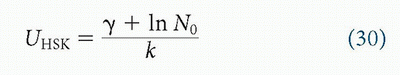Where: UHSK = mean time until sterility

Υ = Euler’s constant (0.5772)

k = reaction rate constant

Converting this to base 10 logarithms and replacing k with DT (see discussion under equation ), we arrive at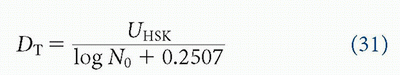Using equation (31), if the UHSK is known, in addition to the initial number of microorganisms (N0) the DT value can be estimated.

When ri is the number of sterile replicates out of n; heated to a time Ui, then the HSK estimate of UHSK is given by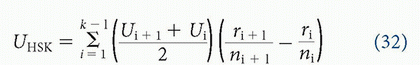where UHSK = HSK heating time estimate (mean time until sterility)

Uk = first heating time with all units negative

Ui = ith heating time

ri = number of replicate units negative at Ui

ni = total number of replicate units heated at Ui

If successive times differ by a constant d and the same number of replicates n is heated at all times (this is known as the Limited Holcomb-Spearman-Karber method), we can write equation (32) as follows:where UHSK = HSK heating time estimate (mean time until sterility)

Uk = first heating time with all units negative

d = time between exposures (should be identical)

n = number of replicates at each exposure interval (should be identical)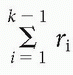= the sum of negative cultures following the last heating time at which all cultures are positive for growth (U2) and Uk – 1

The estimated variance (V) of UHSK assuming equal times between exposure periods and the same number of replicates per exposure period is given by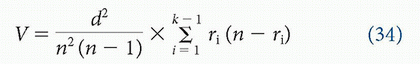The 95% CI for the mean D-value is calculated from the variance of UHSK as follows:

D lower CL: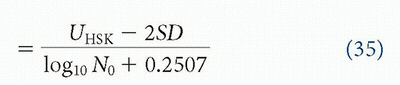TABLE 11.2 Hypothetical fraction-negative dataa

 Heating Interval (Ui) No. of Samples Tested No. of Samples Sterile 7 min 20 0 8 min 20 0 9 min 20 2 10 min 20 8 11 min 20 20 12 min 20 20 aHypothetical inactivation data of a biological indicator (BI) inoculated with a population of 1.5 × 106 spores of Geobacillus stearothermophilus processed in a moist heat resistometer at 121°C.

D upper CL:Based on the earlier discussion and the data in Table 11.2, it is a simple exercise to calculate the D-value for a hypothetical BI with a population of 1.5 × 106 spores per carrier processed at a temperature of 121°C using both the Stumbo-Murphy-Cochran and the Limited Holcomb-Spearman-Karber method as follows:

1. Stumbo-Murphy-Cochran method:Because there are two heating time intervals with fraction-negative results, a DT value is calculated for each fraction-negative interval and averaged together as follows: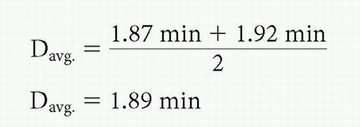2. Limited Holcomb-Spearman-Karber method

First designate the appropriate terms of the method as follows with respect to the data:

n = number of specimens per group (20)

d = the difference in minutes between adjacent exposure times (1 min)

U1 = response of all specimens showing growth in the shortest exposure time adjacent to a dichotomous response (8 min)

Uk = response of all specimens showing no growth in the longest exposure time adjacent to a dichotomous response (11 min)

Where:Calculate the mean heating time until sterility (UHSK) using equation (33):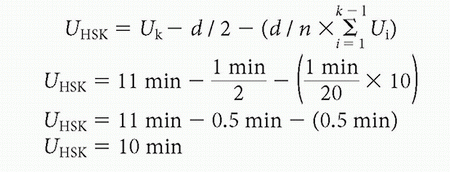Calculate the D-value using equation (31):Calculate the variance of UHSK (VT) as follows: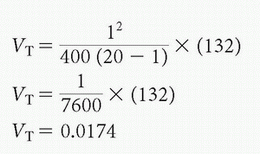Calculate the standard deviation: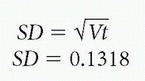Calculate the lower and upper 95% CL for D: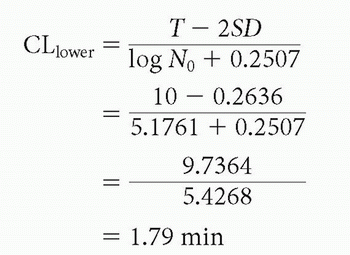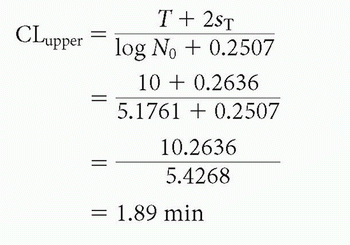Although there may be sound reasons for selecting one form of quantal D-value analysis over another, depending on the application and nature of the data, experience suggests that under well-controlled conditions, the differences between D-values calculated using either the Stumbo-Murphy-Cochran or the Holcomb-Spearman-Karber method are not that significant when the data is suitable for calculation by both methods. A review of the D-values calculated for 30 lots of BIs processed in a moist heat resistometer using both methods suggests that there is little difference in the resulting D-values (<10%) regardless of the method of calculation employed (Table 11.3). Of the two methods, the Holcomb-Spearman-Karber method is generally assumed to be the most rigorous because one can estimate the mean time until sterility (UHSK) and corresponding CIs based solely on the quantal data without knowledge of the initial population or shape of the survivor curve. This in turn can be used to compare the effect of various treatment variables, although calculation of the DT value obviously requires that the initial population be known. Where data are limited, however, the Stumbo-Murphy-Cochran method can provide a reasonable estimation of the efficacy of the treatment conditions. For estimation of the D-value both the Stumbo-Murphy-Cochran and Holcomb-Spearman-Karber methods are subject to any
errors associated with the determination of the initial population (N0) or nonlinearity of the survivor curve. It is also assumed that for each exposure interval, the replicate samples are processed under identical conditions. When replicate samples are processed under ideal conditions in a resistometer (or essentially tightly controlled conditions) as in Table 11.3, these assumptions usually hold and the resulting errors are of a small magnitude such that either method (Stumbo-Murphy-Cochran or Holcomb-Spearman-Karber) will provide an acceptable result.

TABLE 11.3 Comparison of D121°C-values of moist heat biological indicators calculated by both the Stumbo-Murphy-Cochran and Limited Holcomb-Spearman-Karber methods

 BI type Labeled D121°C-Value LL UL SMC D121°C-Value LHSK D121°C-Value Paper strip 1.7 1.36 2.04 1.9 1.9 Paper strip 2.0 1.6 2.4 1.9 1.8 Paper strip 2.1 1.68 2.52 1.8 1.8 Paper strip 1.9 1.52 2.28 1.7 1.7 Paper strip 1.8 1.44 2.16 1.6 1.6 Paper strip 1.9 1.52 2.28 1.6 1.6 Paper strip 2.4 1.92 2.88 2.4 2.4 Paper strip 1.5 1.2 1.8 1.8 1.8 Paper strip 2.2 1.76 2.64 1.9 1.9 Paper strip 1.7 1.36 2.04 1.9 1.9 Self-contained 2.1 1.68 2.52 2.1 2.1 Self-contained 2.1 1.68 2.52 2.3 2.3 Self-contained 1.9 1.52 2.28 2.2 2.2 Self-contained 2.1 1.68 2.52 2.1 2.1 Self-contained 2.1 1.68 2.52 2.1 2.1 Self-contained 1.7 1.36 2.04 1.8 1.7 Self-contained 1.7 1.36 2.04 1.8 1.8 Self-contained 2.0 1.6 2.4 1.9 2.0 Self-contained 1.8 1.44 2.16 1.8 1.9 Self-contained 1.7 1.36 2.04 1.5 1.5 Ampule 1.8 1.44 2.16 1.7 1.7 Ampule 1.7 1.36 2.04 1.8 1.8 Ampule 2.1 1.68 2.52 2.2 2.2 Ampule 2.0 1.6 2.4 2.3 2.3 Ampule 1.8 1.44 2.16 1.8 1.8 Ampule 1.9 1.52 2.28 1.9 1.7 Ampule 1.9 1.52 2.28 1.8 1.8 Ampule 1.9 1.52 2.28 1.9 1.9 Ampule 2.3 1.84 2.76 2.5 2.4 Ampule 1.7 1.36 2.04 1.5 1.5 Abbreviations: BI, biological indicator; LHSK, Limited Holcomb-Spearman-Karber method; LL, lower limit (labeled D-value −20%); SMC, Stumbo-Murphy-Cochran method; UL, upper limit (labeled D-value +20%).STERILITY ASSURANCE LEVEL

Thus far, we have presented an analysis of the semilogarithmic model of the inactivation of microorganisms based on modeling performed in the direct
enumeration (plate count) and quantal or fraction-negative regions of the survivor curve. From this data, we can then predict that region of the survivor curve where we would expect to recover less than 0.01 or 0.001 surviving microorganisms. This region (known as the total kill region—see Figure 11.6) represents the practical limit of measurement of the survivor curve using current methodology because the use of a significant number of replicates (ie, BIs or products inoculated with the test organism) is required. Confidence in and correlation of the data provided by total kill analysis coupled with the data generated in the direct enumeration and quantal or fraction-negative analysis regions of the survivor curve allows us to extend the processing time to a desired probability of a surviving microorganism or sterility assurance level (SAL), which is typically 12D or 10-6 for most sterilization applications (see Figure 11.3). This relationship is generally well established for most moist heat sterilization processing conditions over a temperature range of 110°C to 135°C and dry heat sterilization conditions of 160°C to 190°C and is recognized in many international pharmacopeias and standards as overkill or conservative processing conditions as described in Table 11.4. Note that there is nothing magical about an SAL of 10-6. This is simply a level of probability (one-in-a-million) that provided a conservative level in risk assessments and was apparently established during the 1960s with the development of guidelines for animal safety testing.55 Other SALs such as 10-3 may be perfectly acceptable depending on the application, such as in the past with products that contact intact skin or mucosa such as electrocardiogram electrodes or examination gloves. Overall, alternative SALs to demonstrate sterility may be acceptable when associated with a rigorous risk assessment.56 If we have established the D-value of a suitable test organism (typically spores of G stearothermophilus for moist heat sterilization and spores of B atrophaeus for dry heat sterilization conditions) based on both the survivor curve method and quantal or fraction-negative method and demonstrated reasonably good agreement between the predicted D-values, one can determine the desired sterilization value (FT) as follows:

TABLE 11.4 Typical overkill or conservative heat processing conditions

 Conditions Minimum BI D-Value (ISO) Temperature 12D Hold Timea Typical Cycles Moist heat 1.5 min 121°C 121°C 18 min Gravity displacement: 30 minb 132°C 1.4 minc Prevacuum: 4 minb Dry heat 2.0 min 160°C 160°C 24 min 120 mind 180°C 2.4 min 30 mine 250°C NAf 30 min (depyrogenation)g a USP <1221>. Parenteral Drug Association (PDA) Technical Monograph No. 1 2007. Theoretical hold time based on 12D reduction. Actual sterilization exposure times should be determined by validation.b Typical preprogrammed health care cycles that include allowance for lag time with standard loads.53 Liquid loads may exhibit significant temperature lag depending on container configuration and volume (Joslyn, 1991).c Estimated based on a z-value of 10°C. Actual hold time may vary depending on construction of biological indicator (BI) (Joslyn, 1991).d Data from Joslyn.58e Data from Hancock.54f NA, not applicable. Validation based on 3 log reduction of endotoxin challenge.g Data from Williams.184Where: FT = sterilization value at temperature T

DT = D-value at temperature T

SLR = spore log reduction

Note that this term is also frequently referenced as FBIO, where FBIO = D (log N0 – log N). In those instances, where the DT value predicted by the survivor curve method and quantal or fraction-negative method are different, prudence would suggest that the higher estimate would be employed to determine FT or FBIO.

Assuming one desires to attain an SAL of 10-6, this would correlate to 12 log reduction of the appropriate test organism if considering a worst case starting population of 106. If we have documented a D-value of 1.5 minutes, the minimum exposure time or sterilization
value necessary to achieve an SAL of 10-6 is easily calculated as FT = (1.5 min) (12) = 18 minutes. Although this provides a sound theoretical basis for the initial sterilization process design, further work is necessary to confirm that the sterilization processing conditions within the product and load are within the desired range. It may also be necessary to establish the relationship between the resistance of the test organism employed for process development and validation and the resistance of the microorganisms present on or with the product (product bioburden). For most thermal processing conditions, it is usually accepted that the resistance of the reference test organism exceeds that of most common forms of product bioburden, but this assumption should be verified particularly for applications where product may have been exposed to microorganisms of unusually high number or heat resistance, such as prions. In these circumstances, further pretreatment or extended thermal processing conditions may be necessary to render the product or goods safe for use.THE TEMPERATURE COEFFICIENT MODEL OF MICROBIAL DESTRUCTION

In the earlier discussion, we have assumed that temperature has remained relatively constant. In reality, this is often not the case. As the astute reader well knows under most thermal processing conditions, the goods or items being processed may exhibit a significant temperature lag with the temperatures within the chamber because they are being heated to the desired exposure conditions. Further fluctuations of the temperature within the load will occur during the exposure phase of the process because the chamber temperatures fluctuate about the temperature set point with a further lag in load temperature at the end of the process as temperature of the chamber returns to ambient. Depending on the nature of the load and processing conditions, the variation in temperature between the load and the chamber may be significant. Thus, how is one to resolve the ideal processing conditions discussed earlier (exposure at a fixed temperature for a fixed time) with the realities of real-world processing conditions? Fortuitously, just as a linear relationship exists between exposure time and the inactivation of microorganisms under constant temperature conditions (the semilogarithmic model), a linear relationship also exists between the rate of inactivation of microorganisms (the D-value) and variation in temperature. This relationship (known as the temperature coefficient or z-value) is also of a semilogarithmic nature.4 As seen in Figure 11.8, a plot of the logarithm of the D-value at various temperatures versus temperature on an arithmetic scale generally results in a straight line, the negative reciprocal of the slope of which is known as the z-value, or the change in temperature required for a 90% or 1-log change in the D-value. Note that this relationship holds regardless of whether one plots the logarithm of the D-value or the F-value (D [log N0 – log N]) as both the thermal resistance curve (log D versus temperature) and thermal death time curve (log F versus temperature) parallel one another. An estimate of the z-value can be obtained as follows when data is available at two different temperatures: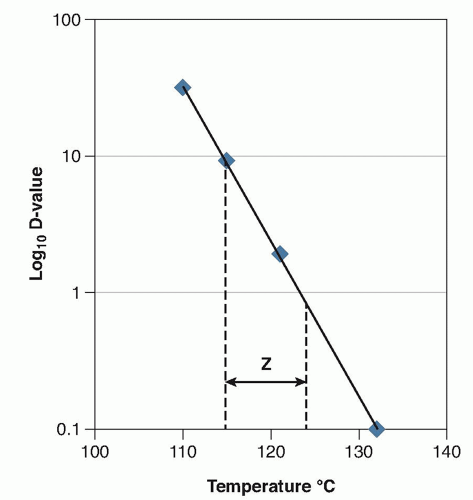FIGURE 11.8 Plot of the z-value of a paper strip biological indicator processed in a moist heat resistometer over a temperature range of 115°C to 132°C. z = 8.9°C.Generally, determination of the z-value should include measurements of the D-value or F-value made over at least three and preferably four different temperatures with the z-value determined by linear regression analysis of a plot of the log of the D- or F-value versus temperature. For moist heat applications, temperatures ranging from 110°C to 138°C are typical,11 whereas temperatures of 150°C to 180°C are most often employed for dry heat applications.12 The goodness of fit of the data can then be evaluated from determination of the coefficient of determination, r2.11 Pflug et al23 have presented a detailed discussion of the correlation of the Bigelow z-value model with the Arrhenius model of reaction rate kinetics and shown that both methods of analysis are essentially
equivalent with the Q10 value (the change in the reaction rate constant k for a 10°C change in temperature, typically a value of 2 for many chemical and biological reactions) being related to the z-value as follows: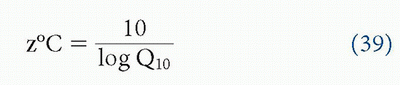For most calculations related to moist heat processing, a z-value of 10°C (18°F) based on the thermal resistance of bacterial spores is employed, although z-values reported in the literature have ranged from as low as 1°C to as high as 21°C for various microorganisms.57 For dry heat processing conditions, a z-value of 20°C (38°F) is assumed as dry heat is less efficacious than moist heat regarding the inactivation of microorganisms at any given temperature. Some of the discrepancy in reported z-values may be attributed to variations in experimental conditions or other factors. Of particular importance is the menstruum in which the test organisms are suspended and also the construction of the test organism system. Whereas for moist heat sterilization, paper strip BIs consisting of paper strip carriers on which a suspension of spores of G stearothermophilus is deposited and packaged within a glassine envelope typically display consistent z-values in the range of 9°C to 11°C, self-contained BIs may exhibit different z-values due to the effect of thermal lag.58 For most commercially available BIs employed for process development and validation, a minimum z-value of 6°C is required for application with moist heat sterilization11 and a minimum z-value of 20°C is required for application with dry heat sterilization processes.12 Note that BIs are best employed under the same conditions as which they have been qualified; otherwise, the results may be misleading.

Only gold members can continue reading. Log In or Register to continue

May 9, 2021 | Posted by in MICROBIOLOGY | Comments Off on Microbial Inactivation Kinetics and Heat Disinfection
Premium Wordpress Themes by UFO Themes